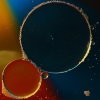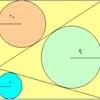# Resources tagged with: Regular polygons and circles

Filter by: Content type:
Age range:
Challenge level:

### There are 67 results

Broad Topics > Angles, Polygons, and Geometrical Proof > Regular polygons and circles##### Age 16 to 18 Challenge Level:

A circle is inscribed in an equilateral triangle. Smaller circles touch it and the sides of the triangle, the process continuing indefinitely. What is the sum of the areas of all the circles?### Crescents and Triangles

##### Age 14 to 16 Challenge Level:

Can you find a relationship between the area of the crescents and the area of the triangle?### Encircling

##### Age 14 to 16 Challenge Level:

An equilateral triangle is sitting on top of a square. What is the radius of the circle that circumscribes this shape?### Salinon

##### Age 14 to 16 Challenge Level:

This shape comprises four semi-circles. What is the relationship between the area of the shaded region and the area of the circle on AB as diameter?### Circle Packing

##### Age 14 to 16 Challenge Level:

Equal circles can be arranged so that each circle touches four or six others. What percentage of the plane is covered by circles in each packing pattern? ...##### Age 14 to 16 Challenge Level:

The sides of a triangle are 25, 39 and 40 units of length. Find the diameter of the circumscribed circle.### Fitting In

##### Age 14 to 16 Challenge Level:

The largest square which fits into a circle is ABCD and EFGH is a square with G and H on the line CD and E and F on the circumference of the circle. Show that AB = 5EF. Similarly the largest. . . .### Semi-detached

##### Age 14 to 16 Challenge Level:

A square of area 40 square cms is inscribed in a semicircle. Find the area of the square that could be inscribed in a circle of the same radius.### Some(?) of the Parts

##### Age 14 to 16 Challenge Level:

A circle touches the lines OA, OB and AB where OA and OB are perpendicular. Show that the diameter of the circle is equal to the perimeter of the triangle### Holly

##### Age 14 to 16 Challenge Level:

The ten arcs forming the edges of the "holly leaf" are all arcs of circles of radius 1 cm. Find the length of the perimeter of the holly leaf and the area of its surface.### The Pillar of Chios

##### Age 14 to 16 Challenge Level:

Semicircles are drawn on the sides of a rectangle. Prove that the sum of the areas of the four crescents is equal to the area of the rectangle.### Circumspection

##### Age 14 to 16 Challenge Level:

M is any point on the line AB. Squares of side length AM and MB are constructed and their circumcircles intersect at P (and M). Prove that the lines AD and BE produced pass through P.##### Age 14 to 16 Challenge Level:

Make five different quadrilaterals on a nine-point pegboard, without using the centre peg. Work out the angles in each quadrilateral you make. Now, what other relationships you can see?### Curvy Areas

##### Age 14 to 16 Challenge Level:

Have a go at creating these images based on circles. What do you notice about the areas of the different sections?### Arclets Explained

##### Age 11 to 16

This article gives an wonderful insight into students working on the Arclets problem that first appeared in the Sept 2002 edition of the NRICH website.### Partly Circles

##### Age 14 to 16 Challenge Level:

What is the same and what is different about these circle questions? What connections can you make?##### Age 14 to 16 Challenge Level:

Given a square ABCD of sides 10 cm, and using the corners as centres, construct four quadrants with radius 10 cm each inside the square. The four arcs intersect at P, Q, R and S. Find the. . . .### Tricircle

##### Age 14 to 16 Challenge Level:

The centre of the larger circle is at the midpoint of one side of an equilateral triangle and the circle touches the other two sides of the triangle. A smaller circle touches the larger circle and. . . .### LOGO Challenge - Following On

##### Age 11 to 18 Challenge Level:

Remember that you want someone following behind you to see where you went. Can yo work out how these patterns were created and recreate them?### Semi-square

##### Age 14 to 16 Challenge Level:

What is the ratio of the area of a square inscribed in a semicircle to the area of the square inscribed in the entire circle?### The Medieval Octagon

##### Age 14 to 16 Challenge Level:

Medieval stonemasons used a method to construct octagons using ruler and compasses... Is the octagon regular? Proof please.### Three Four Five

##### Age 14 to 16 Challenge Level:

Two semi-circles (each of radius 1/2) touch each other, and a semi-circle of radius 1 touches both of them. Find the radius of the circle which touches all three semi-circles.### Like a Circle in a Spiral

##### Age 7 to 16 Challenge Level:

A cheap and simple toy with lots of mathematics. Can you interpret the images that are produced? Can you predict the pattern that will be produced using different wheels?### Ford Circles

##### Age 16 to 18 Challenge Level:

Can you find the link between these beautiful circle patterns and Farey Sequences?### Get Cross

##### Age 14 to 16 Challenge Level:

A white cross is placed symmetrically in a red disc with the central square of side length sqrt 2 and the arms of the cross of length 1 unit. What is the area of the disc still showing?### From All Corners

##### Age 14 to 16 Challenge Level:

Straight lines are drawn from each corner of a square to the mid points of the opposite sides. Express the area of the octagon that is formed at the centre as a fraction of the area of the square.### Escriptions

##### Age 16 to 18 Challenge Level:

For any right-angled triangle find the radii of the three escribed circles touching the sides of the triangle externally.### Lunar Angles

##### Age 16 to 18 Challenge Level:

What is the sum of the angles of a triangle whose sides are circular arcs on a flat surface? What if the triangle is on the surface of a sphere?### Squaring the Circle and Circling the Square

##### Age 14 to 16 Challenge Level:

If you continue the pattern, can you predict what each of the following areas will be? Try to explain your prediction.### LOGO Challenge - Circles as Animals

##### Age 11 to 16 Challenge Level:

See if you can anticipate successive 'generations' of the two animals shown here.### Area I'n It

##### Age 16 to 18 Challenge Level:

Triangle ABC has altitudes h1, h2 and h3. The radius of the inscribed circle is r, while the radii of the escribed circles are r1, r2 and r3 respectively. Prove: 1/r = 1/h1 + 1/h2 + 1/h3 = 1/r1 +. . . .### Just Touching

##### Age 16 to 18 Challenge Level:

Three semi-circles have a common diameter, each touches the other two and two lie inside the biggest one. What is the radius of the circle that touches all three semi-circles?### Polycircles

##### Age 14 to 16 Challenge Level:

Show that for any triangle it is always possible to construct 3 touching circles with centres at the vertices. Is it possible to construct touching circles centred at the vertices of any polygon?##### Age 14 to 16 Challenge Level:

Investigate the properties of quadrilaterals which can be drawn with a circle just touching each side and another circle just touching each vertex.### Circles in Circles

##### Age 16 to 18 Challenge Level:

This pattern of six circles contains three unit circles. Work out the radii of the other three circles and the relationship between them.### Sangaku

##### Age 16 to 18 Challenge Level:

The square ABCD is split into three triangles by the lines BP and CP. Find the radii of the three inscribed circles to these triangles as P moves on AD.### Efficient Packing

##### Age 14 to 16 Challenge Level:

How efficiently can you pack together disks?### Pent

##### Age 14 to 18 Challenge Level:

The diagram shows a regular pentagon with sides of unit length. Find all the angles in the diagram. Prove that the quadrilateral shown in red is a rhombus.### LOGO Challenge 1 - Star Square

##### Age 7 to 16 Challenge Level:

Can you use LOGO to create this star pattern made from squares. Only basic LOGO knowledge needed.### Using Geogebra

##### Age 11 to 18### Incircles Explained

##### Age 16 to 18### Gold Yet Again

##### Age 16 to 18 Challenge Level:

Nick Lord says "This problem encapsulates for me the best features of the NRICH collection."### Dodecawhat

##### Age 14 to 16 Challenge Level:

Follow instructions to fold sheets of A4 paper into pentagons and assemble them to form a dodecahedron. Calculate the error in the angle of the not perfectly regular pentagons you make.### F'arc'tion

##### Age 14 to 16 Short Challenge Level:

At the corner of the cube circular arcs are drawn and the area enclosed shaded. What fraction of the surface area of the cube is shaded? Try working out the answer without recourse to pencil and. . . .### Star Gazing

##### Age 14 to 16 Challenge Level:

Find the ratio of the outer shaded area to the inner area for a six pointed star and an eight pointed star.### Tessellation Interactivity

##### Age 7 to 16 Challenge Level:

An environment that enables you to investigate tessellations of regular polygons### The Dodecahedron

##### Age 16 to 18 Challenge Level:

What are the shortest distances between the centres of opposite faces of a regular solid dodecahedron on the surface and through the middle of the dodecahedron?### Orthogonal Circle

##### Age 16 to 18 Challenge Level:

Given any three non intersecting circles in the plane find another circle or straight line which cuts all three circles orthogonally.### Logosquares

##### Age 16 to 18 Challenge Level:

Ten squares form regular rings either with adjacent or opposite vertices touching. Calculate the inner and outer radii of the rings that surround the squares.### First Forward Into Logo 4: Circles

##### Age 7 to 16 Challenge Level:

Learn how to draw circles using Logo. Wait a minute! Are they really circles? If not what are they?